# Simply Scalloped Mats Thru the Year

 Showing 1 - 24 of 24 results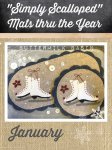View Large Image
Simply Scalloped Mats Thru the Year - January Pattern

The January Simply Scalloped Mat pattern gives you the instructions to make both the oval mat and circle mat with ice skates. The oval mat is 7" x 13", and the round mat is 7.5" round.

 Price \$ 7.95 Quantity: 0123456789101112131415161718192021222324252627282930313233343536373839404142434445464748495051525354555657585960616263646566676869707172737475767778798081828384858687888990919293949596979899100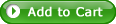View Large Image
Simply Scalloped Mats Thru the Year - January Pattern and Kit for both Oval Mat and Round Mat

The January Simply Scalloped Mat pattern and kit for both the oval mat and the round mat includes all of the wool you will need to make both mats, with fabric backings. The oval mat is 7" x 13" and the round mat is 7.5" round.

 Price \$ 36.95 Quantity: 0123456789101112131415161718192021222324252627282930313233343536373839404142434445464748495051525354555657585960616263646566676869707172737475767778798081828384858687888990919293949596979899100View Large Image
Simply Scalloped Mats Thru the Year - February Pattern

The February Simply Scalloped Mat pattern gives you the instructions to make both the oval mat and circle mat with ice skates. The oval mat is 7" x 13", and the round mat is 7.5" round.

 Price \$ 7.95 Quantity: 0123456789101112131415161718192021222324252627282930313233343536373839404142434445464748495051525354555657585960616263646566676869707172737475767778798081828384858687888990919293949596979899100View Large Image
Simply Scalloped Mats Thru the Year - February Pattern and Kit for both Oval Mat and Round Mat

The February Simply Scalloped Mat pattern and kit for both the oval mat and the round mat includes all of the wool you will need to make both mats, with fabric backings. The oval mat is 7" x 13" and the round mat is 7.5" round.

 Price \$ 36.95 Quantity: 0123456789101112131415161718192021222324252627282930313233343536373839404142434445464748495051525354555657585960616263646566676869707172737475767778798081828384858687888990919293949596979899100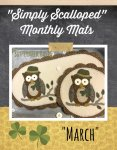View Large Image
Simply Scalloped Mats Thru the Year - March Pattern

The March Simply Scalloped Mat pattern gives you the instructions to make both the oval mat and circle mat. The oval mat is 7" x 13", and the round mat is 7.5" round.

 Price \$ 7.95 Quantity: 0123456789101112131415161718192021222324252627282930313233343536373839404142434445464748495051525354555657585960616263646566676869707172737475767778798081828384858687888990919293949596979899100View Large Image
Simply Scalloped Mats Thru the Year - March Kit & Pattern for both Oval Mat & Round Mat

The March Simply Scalloped Mat pattern gives you the instructions to make both the oval mat and circle mat, and this kit includes wool to make both the oval mat and the round mat. The oval mat is 7" x 13", and the round mat is 7.5" round.

 Price \$ 36.95 Quantity: 0123456789101112131415161718192021222324252627282930313233343536373839404142434445464748495051525354555657585960616263646566676869707172737475767778798081828384858687888990919293949596979899100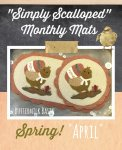View Large Image
Simply Scalloped Mats Thru the Year - April Pattern

The April Simply Scalloped Mat pattern gives you the instructions to make both the oval mat and circle mat with a chick and egg! The oval mat is 7" x 13", and the round mat is 7.5" round.

 Price \$ 7.95 Quantity: 0123456789101112131415161718192021222324252627282930313233343536373839404142434445464748495051525354555657585960616263646566676869707172737475767778798081828384858687888990919293949596979899100View Large Image
Simply Scalloped Mats Thru the Year - April Kit & Pattern for both Oval Mat & Round Mat

The April Simply Scalloped Mat pattern gives you the instructions to make both the oval mat and circle mat, and this kit includes wool to make both the oval mat and the round mat. The oval mat is 7" x 13", and the round mat is 7.5" round.

 Price \$ 36.95 Quantity: 0123456789101112131415161718192021222324252627282930313233343536373839404142434445464748495051525354555657585960616263646566676869707172737475767778798081828384858687888990919293949596979899100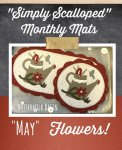View Large Image
Simply Scalloped Mats Thru the Year - May Pattern

The May Simply Scalloped Mat pattern gives you the instructions to make both the oval mat and circle mat with a flowering can and flowers! The oval mat is 7" x 13", and the round mat is 7.5" round.

 Price \$ 7.95 Quantity: 0123456789101112131415161718192021222324252627282930313233343536373839404142434445464748495051525354555657585960616263646566676869707172737475767778798081828384858687888990919293949596979899100View Large Image
Simply Scalloped Mats Thru the Year - May Kit & Pattern for both Oval Mat & Round Mat

The May Simply Scalloped Mat pattern gives you the instructions to make both the oval mat and circle mat, and this kit includes wool to make both the oval mat and the round mat. The oval mat is 7" x 13", and the round mat is 7.5" round.

 Price \$ 36.95 Quantity: 012345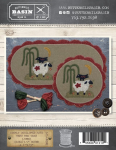View Large Image
Simply Scalloped Mats Thru the Year - June Pattern

The June Simply Scalloped Mat pattern gives you the instructions to make both the oval mat and circle mat. The round mat is 7.5" round and the oval mat is 7" x 13".

 Price \$ 7.95 Quantity: 0123456789101112131415161718192021222324252627282930313233343536373839404142434445464748495051525354555657585960616263646566676869707172737475767778798081828384858687888990919293949596979899100View Large Image
Simply Scalloped Mats Thru the Year - June Kit & Pattern for both Oval Mat & Round Mat

The June Simply Scalloped Mat pattern gives you the instructions to make both the oval mat and circle mat, and this kit includes wool to make both the oval mat and the round mat. The oval mat is 7" x 13", and the round mat is 7.5" round.

 Price \$ 36.95 Quantity: 0123456789101112131415161718192021222324252627282930313233343536373839404142434445464748495051525354555657585960616263646566676869707172737475767778798081828384858687888990919293949596979899100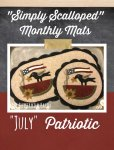View Large Image
Simply Scalloped Mats Thru the Year - July Pattern

The July Simply Scalloped Mat pattern gives you the instructions to make both the oval mat and circle mat. The oval mat is 7" x 13", and the round mat is 7.5" round.

 Price \$ 7.95 Quantity: 0123456789101112131415161718192021222324252627282930313233343536373839404142434445464748495051525354555657585960616263646566676869707172737475767778798081828384858687888990919293949596979899100View Large Image
Simply Scalloped Mats Thru the Year - July Pattern & Kit for both Oval Mat & Round Mat

The July Simply Scalloped Mat pattern gives you the instructions to make both the oval mat and circle mat, and this kit includes wool to make both the oval mat and the round mat. The oval mat is 7" x 13", and the round mat is 7.5" round.

 Price \$ 36.95 Quantity: 0123456789101112131415161718192021222324252627282930313233343536373839404142434445464748495051525354555657585960616263646566676869707172737475767778798081828384858687888990919293949596979899100View Large Image
Simply Scalloped Mats Thru the Year - August Pattern

The August Simply Scalloped Mat pattern gives you the instructions to make both the oval mat and circle mat. The oval mat is 7" x 13" and the round mat is 7.5" round.

 Price \$ 7.95 Quantity: 0123456789101112131415161718192021222324252627282930313233343536373839404142434445464748495051525354555657585960616263646566676869707172737475767778798081828384858687888990919293949596979899100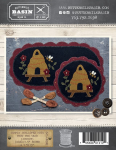View Large Image
Simply Scalloped Mats Thru the Year - August Pattern & Kit for both Oval Mat & Round Mat

The August Simply Scalloped Mat pattern gives you the instructions to make both the oval mat and circle mat, and this kit includes wool to make both the oval mat and the round mat. The oval mat is 7" x 13", and the round mat is 7.5" round.

 Price \$ 36.95 Quantity: 0123456789101112131415161718192021222324252627282930313233343536373839404142434445464748495051525354555657585960616263646566676869707172737475767778798081828384858687888990919293949596979899100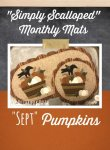View Large Image
Simply Scalloped Mats Thru the Year - September Pattern

The January Simply Scalloped Mat pattern gives you the instructions to make both the oval mat and circle mat with ice skates. The oval mat is 7" x 13", and the round mat is 7.5" round.

 Price \$ 7.95 Quantity: 0123456789101112131415161718192021222324252627282930313233343536373839404142434445464748495051525354555657585960616263646566676869707172737475767778798081828384858687888990919293949596979899100View Large Image
Simply Scalloped Mats Thru the Year - September Pattern & Kit for both Oval Mat & Round Mat

The August Simply Scalloped Mat pattern gives you the instructions to make both the oval mat and circle mat, and this kit includes wool to make both the oval mat and the round mat. The oval mat is 7" x 13", and the round mat is 7.5" round.

 Price \$ 36.95 Quantity: 0123456789101112131415161718192021222324252627282930313233343536373839404142434445464748495051525354555657585960616263646566676869707172737475767778798081828384858687888990919293949596979899100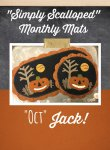View Large Image
Simply Scalloped Mats Thru the Year - October Pattern

The January Simply Scalloped Mat pattern gives you the instructions to make both the oval mat and circle mat with ice skates. The oval mat is 7" x 13", and the round mat is 7.5" round.

 Price \$ 7.95 Quantity: 0123456789101112131415161718192021222324252627282930313233343536373839404142434445464748495051525354555657585960616263646566676869707172737475767778798081828384858687888990919293949596979899100View Large Image
Simply Scalloped Mats Thru the Year - October Pattern & Kit for both Oval Mat & Round Mat

The August Simply Scalloped Mat pattern gives you the instructions to make both the oval mat and circle mat, and this kit includes wool to make both the oval mat and the round mat. The oval mat is 7" x 13", and the round mat is 7.5" round.

 Price \$ 36.95 Quantity: 0123456789101112131415161718192021222324252627282930313233343536373839404142434445464748495051525354555657585960616263646566676869707172737475767778798081828384858687888990919293949596979899100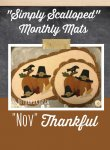View Large Image
Simply Scalloped Mats Thru the Year - November Pattern

The January Simply Scalloped Mat pattern gives you the instructions to make both the oval mat and circle mat with ice skates. The oval mat is 7" x 13", and the round mat is 7.5" round.

 Price \$ 7.95 Quantity: 0123456789101112131415161718192021222324252627282930313233343536373839404142434445464748495051525354555657585960616263646566676869707172737475767778798081828384858687888990919293949596979899100View Large Image
Simply Scalloped Mats Thru the Year - November Pattern & Kit for both Oval Mat & Round Mat

The August Simply Scalloped Mat pattern gives you the instructions to make both the oval mat and circle mat, and this kit includes wool to make both the oval mat and the round mat. The oval mat is 7" x 13", and the round mat is 7.5" round.

 Price \$ 36.95 Quantity: 0123456789101112131415161718192021222324252627282930313233343536373839404142434445464748495051525354555657585960616263646566676869707172737475767778798081828384858687888990919293949596979899100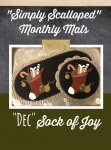View Large Image
Simply Scalloped Mats Thru the Year - December * Pattern

The December Simply Scalloped Mat pattern gives you the instructions to make both the oval mat and circle mat with ice skates. The oval mat is 7" x 13", and the round mat is 7.5" round.

 SALE \$ 7.95  \$ 4.50 Quantity: 0123456789101112131415161718192021222324252627282930313233343536373839404142434445464748495051525354555657585960616263646566676869707172737475767778798081828384858687888990919293949596979899100View Large Image
Simply Scalloped Mats Thru the Year - December Pattern & Kit for both Oval Mat & Round Mat

The August Simply Scalloped Mat pattern gives you the instructions to make both the oval mat and circle mat, and this kit includes wool to make both the oval mat and the round mat. The oval mat is 7" x 13", and the round mat is 7.5" round.

 Price \$ 36.95 Quantity: 0123456789101112131415161718192021222324252627282930313233343536373839404142434445464748495051525354555657585960616263646566676869707172737475767778798081828384858687888990919293949596979899100